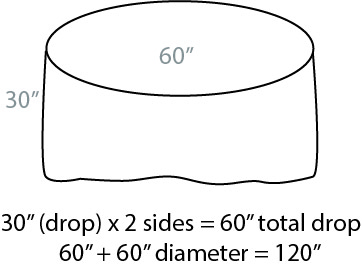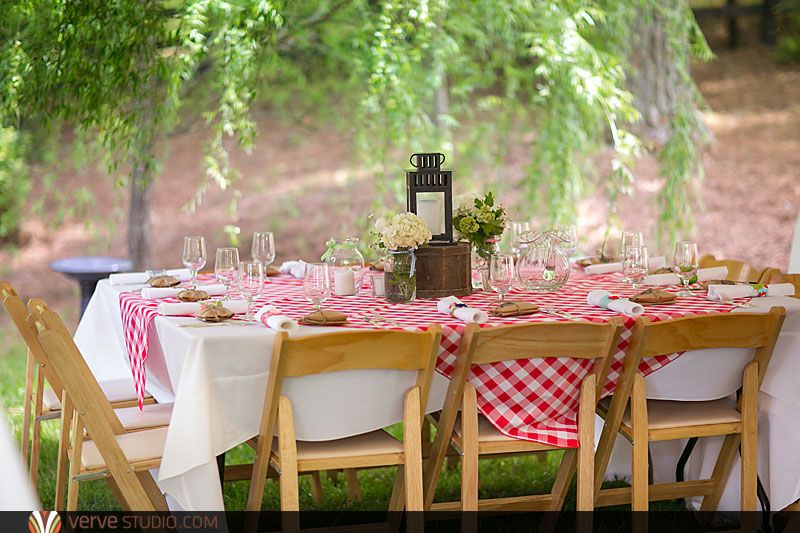## You will need to know two things:Ordering square or rectangle cloths:

Since the drop is on both sides; multiply the drop by 2 and add this measurement to both the width and length.

For example:

if your table is 48 x84″:

for a 12″ drop: multiply 12″ x 2 (24) and add 24 to the width of the table

you will therefore need a 72 x 108″ cloth

Ordering round cloths:

Multiply the drop by 2 and add this measurement to the diameter.

For example: